# Excel WEEKDAY function: get day of week, weekends and workdays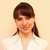by , updated on

If you are looking for an Excel function to get day of week from date, you've landed on the right page. This tutorial will teach you how to use the WEEKDAY formula in Excel to convert a date to a weekday name, filter, highlight and count weekends or workdays, and more.

There are a variety of functions to work with dates in Excel. The day of week function (WEEKDAY) is particularly useful for planning and scheduling, for example to determine the timeframe of a project and automatically remove weekends from the total. So, let's run through the examples one-at-a-time and see how they can help you cope with various date-related tasks in Excel.

## WEEKDAY - Excel function for day of week

The Excel WEEKDAY function is used to return the day of the week from a given date.

The result is an integer, ranging from 1 (Sunday) to 7 (Saturday) by default. If your business logic requires a different enumeration, you can configure the formula to start counting with any other day of week.

The WEEKDAY function is available in all versions of Excel 365 through 2000.

The syntax of the WEEKDAY function is as follows:

WEEKDAY(serial_number, [return_type])

Where:

Serial_number (required) - the date that you want to convert to the weekday number. It can be supplied as a serial number representing the date, as a text string in the format that Excel understands, as a reference to the cell containing the date, or by using the DATE function.

Return_type (optional) - determines what day of the week to use as the first day. If omitted, defaults to the Sun-Sat week.

Here is a list of all supported return_type values:

Return_type Number returned
1 or omitted From 1 (Sunday) to 7 (Saturday)
2 From 1 (Monday) to 7 (Sunday)
3 From 0 (Monday) to 6 (Sunday)
11 From 1 (Monday) to 7 (Sunday)
12 From 1 (Tuesday) to 7 (Monday)
13 From 1 (Wednesday) to 7 (Tuesday)
14 From 1 (Thursday) to 7 (Wednesday)
15 From 1 (Friday) to 7 (Thursday)
16 From 1 (Saturday) to 7 (Friday)
17 From 1 (Sunday) to 7 (Saturday)

Note. The return_type values 11 through 17 were introduced in Excel 2010 and therefore they cannot be used in earlier versions.

## Basic WEEKDAY formula in Excel

For starters, let's see how to use the WEEKDAY formula in its simplest form to get the day number from date.

For example, to get the weekday from date in C4 with the default Sunday - Saturday week, the formula is:

`=WEEKDAY(C4)`

If you have a serial number representing the date (e.g. brought by the DATEVALUE function), you can enter that number directly in the formula:

`=WEEKDAY(45658)`

Also, you can type the date as a text string enclosed in quotation marks directly in the formula. Just be sure to use the date format that Excel expects and can interpret:

`=WEEKDAY("1/1/2025")`

Or, supply the source date in a 100% reliable way using the DATE function:

`=WEEKDAY(DATE(2025, 1,1))`

To use the day mapping other than the default Sun-Sat, enter an appropriate number in the second argument. For example, to start counting days from Monday, the formula is:

`=WEEKDAY(C4, 2)`

In the image below, all the formulas return the day of the week corresponding to January 1, 2025, which is stored as the number 45658 internally in Excel. Depending on the value set in the second argument, the formulas output different results.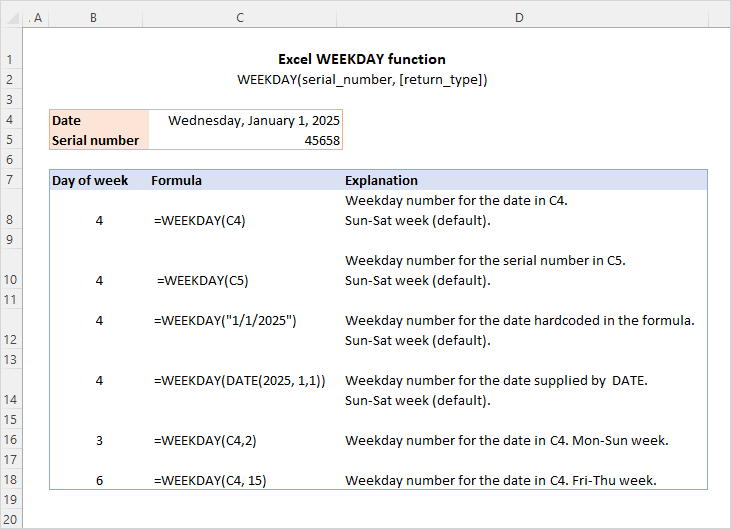At first sight, it may seem that the numbers returned by the WEEKDAY function have very little practical sense. But let's look at it from a different angle and discuss some formulas that solve real-life tasks.

## How to convert Excel date to weekday name

By design, the Excel WEEKDAY function returns the day of the week as a number. To turn the weekday number into the day name, employ the TEXT function.

To get full day names, use the "dddd" format code:

TEXT(WEEKDAY(date), "dddd")

To return abbreviated day names, the format code is "ddd":

TEXT(WEEKDAY(date), "ddd")

For example, to convert the date in A3 to the weekday name, the formula is:

`=TEXT(WEEKDAY(A3), "dddd")`

Or

`=TEXT(WEEKDAY(A3), "ddd")`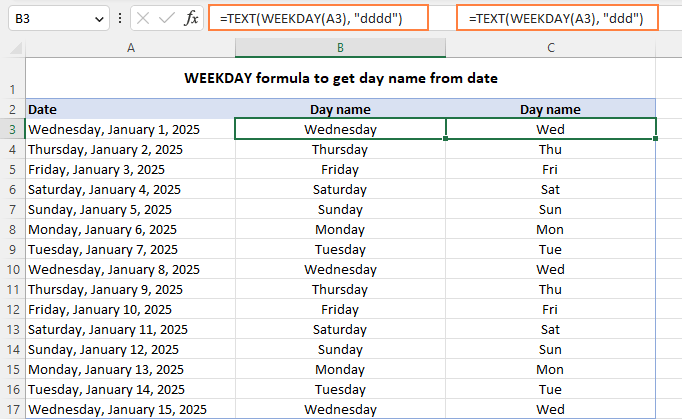Another possible solution is using WEEKDAY together with the CHOOSE function.

For example, to get an abbreviated weekday name from the date in A3, the formula goes as follows:

`=CHOOSE(WEEKDAY(A3),"Sun","Mon","Tus","Wed","Thu","Fri","Sat")`

Here, WEEKDAY returns a serial number from 1 (Sun) to 7 (Sat) and CHOOSE selects the corresponding value from the list. Since the date in A3 (Wednesday) corresponds to 4, CHOOSE outputs "Wed", which is the 4th value in the list.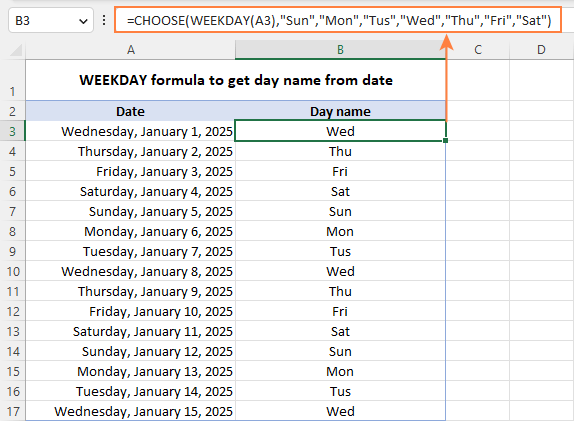Though the CHOOSE formula is slightly more cumbersome to configure, it provides more flexibility letting you output the day names in any format you want. In the above example, we show the abbreviated day names. Instead, you can deliver full names, custom abbreviations or even day names in a different language.

Tip. Another easy way to convert a date to the weekday name is by applying a custom date format. For example, the code format "dddd, mmmm d, yyyy" will have the date displayed as "Friday, January 3, 2025" while "dddd" will show just "Friday".

## Excel WEEKDAY formula to find and filter workdays and weekends

When dealing with a long list of dates, you may want to know which ones are working days and which are weekends.

To identify weekends and weekdays in Excel, build an IF statement with the nested WEEKDAY function. For example:

`=IF(WEEKDAY(A3, 2)<6, "Workday", "Weekend")`

This formula goes to cell A3 and is copied down across as many cells as needed.

In the WEEKDAY formula, you set return_type to 2, which corresponds to the Mon-Sun week where Monday is day 1. So, if the weekday number is less than 6 (Monday through Friday), the formula returns "Workday", otherwise - "Weekend".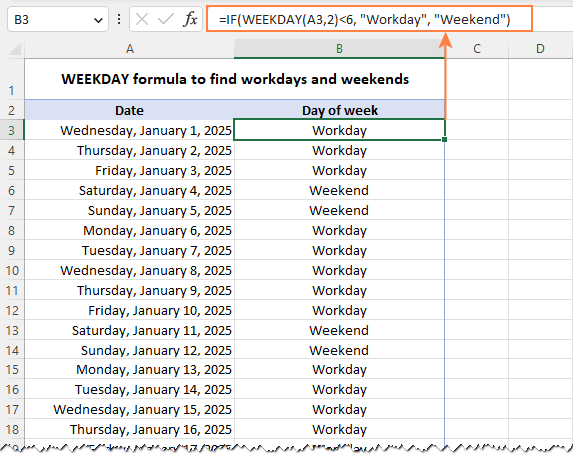To filter weekends or workdays, apply Excel filter to your dataset (Data tab > Filter) and select either "Weekend" or "Workday".

In the screenshot below, we have weekdays filtered out, so only weekends are visible: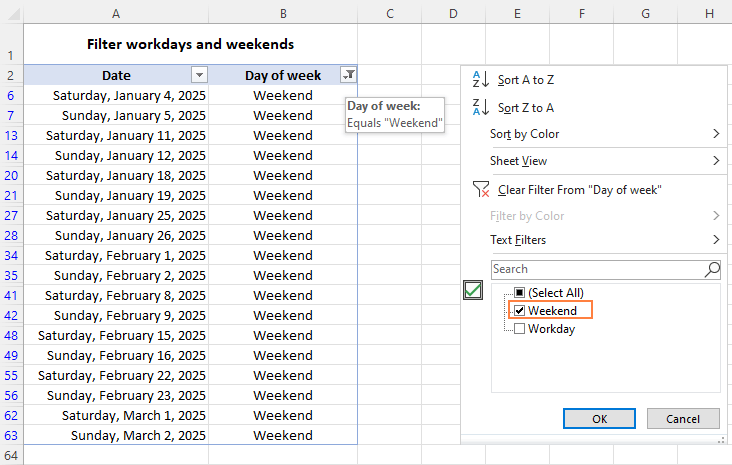If some regional office of your organization works on a different schedule where the days of rest are other than Saturday and Sunday, you can easily adjust the WEEKDAY formula to your needs by specifying a different return_type.

For example, to treat Saturday and Monday as weekends, set return_type to 12, so you'll get the "Tuesday (1) to Monday (7)" week type:

`=IF(WEEKDAY(A2, 12)<6, "Workday", "Weekend")`

## How to highlight weekends workdays and in Excel

To spot weekends and workdays in your worksheet at a glance, you can get them automatically shaded in different colors. For this, use the weekday/weekend formula discussed in the previous example with Excel conditional formatting. As the condition is implied, we only need the core WEEKDAY function without the IF wrapper.

To highlight weekends (Saturday and Sunday):

`=WEEKDAY(\$A2, 2)<6`

To highlight workdays (Monday - Friday):

`=WEEKDAY(\$A2, 2)>5`

Where A2 is the upper-left cell of the selected range.

To set up the conditional formatting rule, the steps are:

1. Select the list of dates (A2:A15 in our case).
2. On the Home tab, in the Styles group, click Conditional formatting > New Rule.
3. In the New Formatting Rule dialog box, select Use a formula to determine which cells to format.
4. In the Format values where this formula is true box, enter the above-mentioned formula for weekends or weekdays.
5. Click the Format button and select the desired format.
6. Click OK twice to save the changes and close the dialog windows.

For the detailed information on each step, please see How to set up conditional formatting with formula.

The result looks pretty nice, doesn't it?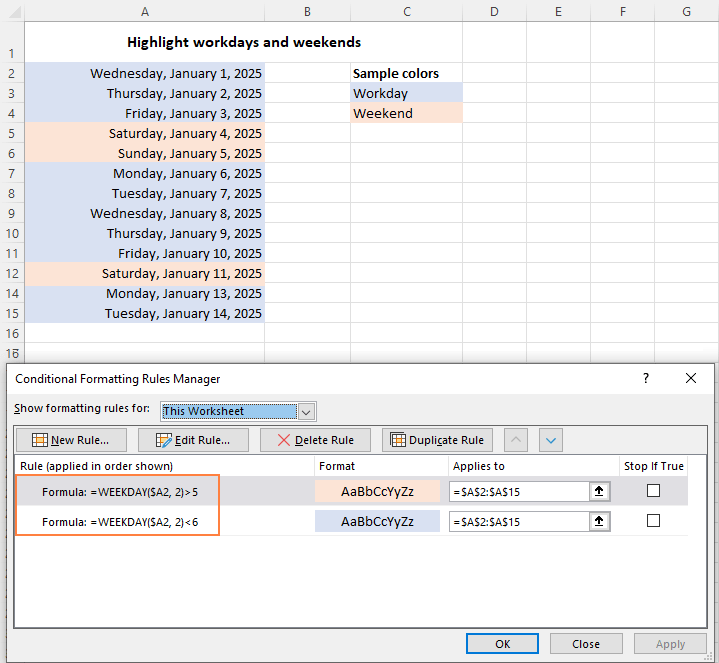## How to count weekdays and weekends in Excel

To get the number of weekdays or weekends in the list of dates, you can use the WEEKDAY function in combination with SUM. For example:

To count weekends, the formula in D3 is:

`=SUM(--(WEEKDAY(A3:A20, 2)>5))`

To count weekdays, the formula in D4 takes this form:

`=SUM(--(WEEKDAY(A3:A20, 2)<6))`

In Excel 365 and Excel 2021 that handle arrays natively, this works as a regular formula as shown in the screenshot below. In Excel 2019 and earlier, press Ctrl + Shift + Enter to make it an array formula.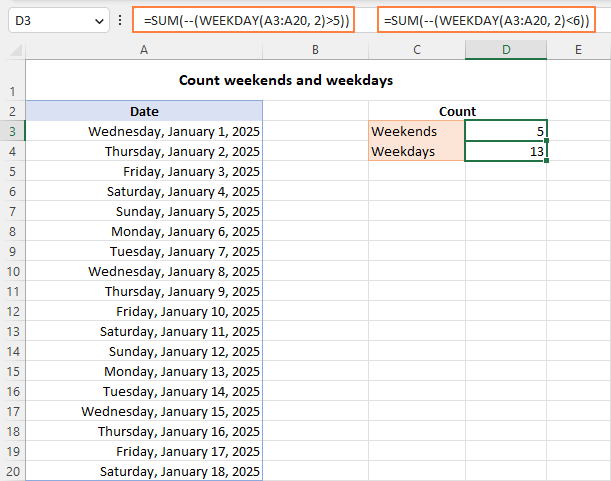How these formulas work:

The WEEKDAY function with return_type set to 2 returns a day number from 1 (Mon) to 7 (Sun) for each date in the range A3:A20. The logical expression checks if the returned numbers are greater than 5 (for weekends) or less than 6 (for weekdays). The result of this operation is an array of TRUE and FALSE values.

The double negation (--) coerces the logical values to 1's and 0's. And the SUM function adds them up. Given that 1 (TRUE) represents the days to be counted and 0 (FALSE) the days to be ignored, you get the desired result.

Tip. To calculate weekdays between two dates, use the NETWORKDAYS or NETWORKDAYS.INTL function.

## If weekday then, if Saturday or Sunday then

Finally, let's discuss a bit more specific case that shows how to determine the day of the week, and if it's Saturday or Sunday then do something, if a weekday then do something else.

IF(WEEKDAY(cell, 2)>5, if_weekend_then, if_weekday_then)

Suppose you are calculating payments for employees who have done some extra work on their days off, so you need to apply different payments rates for workdays and weekends. This can be done using the following IF statement:

• In the logical_test argument, nest the WEEKDAY function that checks whether a given day is a workday or weekend.
• In the value_if_true argument, multiply the number of working hours by the weekend rate (G4).
• In the value_if_false argument, multiply the number of working hours by the workday rate (G3).

The complete formula in D3 takes this form:

`=IF(WEEKDAY(B3, 2)>5, C3*\$G\$4, C3*\$G\$3)`

For the formula to copy correctly to the below cells, be sure to lock the rate cell addresses with the \$ sign (like \$G\$4).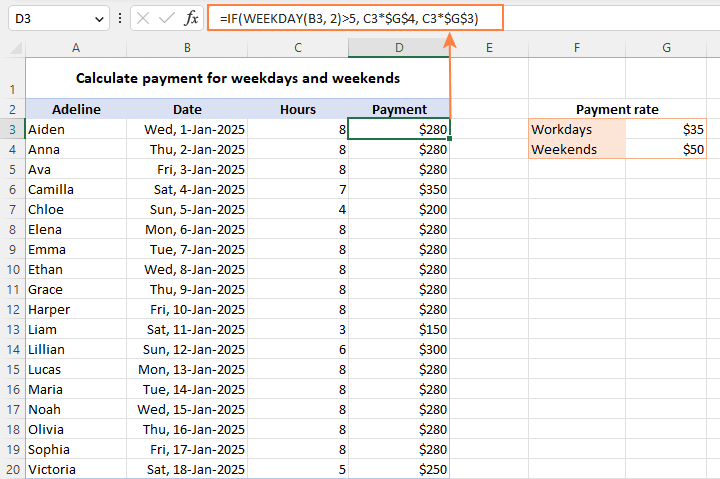## WEEKDAY function not working

Generally, there are two common errors that a WEEKDAY formula may return:

#VALUE! error occurs if either:

• Serial_number or return_type is non-numeric.
• Serial_number is out of supported dates range (1900 to 9999).

#NUM! error occurs when return_type is out of the permitted range (1-3 or 11-17).

This is how to use the WEEKDAY function in Excel to manipulate days of week. In the next article, we will explore Excel functions to operate on bigger time units such as weeks, months and years. Please stay tuned and thank you for reading!